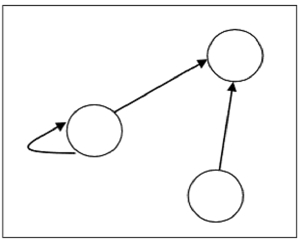# Representation of Relations using Graph

A relation can be represented using a directed graph.

The number of vertices in the graph is equal to the number of elements in the set from which the relation has been defined. For each ordered pair (x, y) in the relation R, there will be a directed edge from the vertex ‘x’ to vertex ‘y’. If there is an ordered pair (x, x), there will be a self- loop on vertex ‘x’.

Suppose, there is a relation R = { (1, 1), (1,2), (3, 2) } on set S = { 1, 2, 3 }, it can be represented by the following graph −Updated on: 26-Aug-2019

1K+ Views